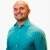# Using the quotient property of logarithms to solve a logarithmic equationThis math lesson will look at an example problem on logs to explain how to use the quotient property of logarithms to solve a logarithmic equation.
3 Views